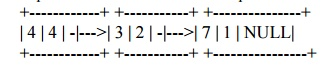Home | | Object Oriented Programming and Data Structures | Polynomial Multiplication Using Linked List

# Polynomial Multiplication Using Linked List

A polynomial expression can be represent as linked list. 4X^4 + 3x^2 + 7X is a polynomial expression and its linked list representation is as follows.

A polynomial expression can be represent as linked list. 4X^4 + 3x^2 + 7X is a polynomial expression and its linked list representation is as follows.Here, each node composed of co-efficient, exponent and a pointer to the next node. Multiplication of polynomial expressions:

3X^3 + 4x^2 + 5X X^4

Output is 3x^7 + 4X^6 + 5X^4

Study Material, Lecturing Notes, Assignment, Reference, Wiki description explanation, brief detail
Object Oriented Programming and Data Structure : Linear Data Structures : Polynomial Multiplication Using Linked List |